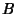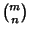## XOR

An operation in Logic known as Exclusive Or. It yields true if exactly one (but not both) of two conditions is true. The Binary XOR operator has the following Truth Table.XORF F F F T T T F T T T F

The Binomial Coefficientmod 2 can be computed using the XOR operationXOR, making Pascal's Triangle mod 2 very easy to construct.

See also And, Binary Operator, Boolean Algebra, Logic, Not, Or, Pascal's Triangle, Truth Table